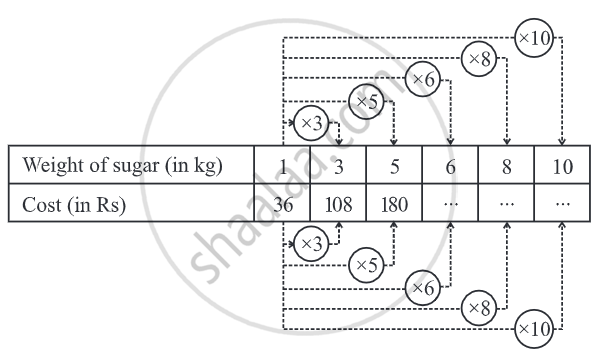# Concept of Direct Proportion

## Definition

Direct Proportion: Two quantities x and y are said to be in direct proportion if they increase or decrease together in such a manner that the ratio of their corresponding values remains constant.

# Direct proportion:

• Two quantities x and y are said to be in direct proportion if they increase or decrease together in such a manner that the ratio of their corresponding values remains constant.

• For example:
i) If the number of articles purchased increases, the total cost also increases.
ii) More the money deposited in a bank, more is the interest earned.

• For example,

In the table below, Let the weight of sugar be x kg and the cost of sugar (in Rs.) be y. As the weight of sugar increases, cost also increases in such a manner that their ratio remains constant. Likewise, if the weight of sugar-reduced, the cost would also reduce. Hence the two variables are in direct proportion.## Relation for Direct Proportion:

• Two quantities x and y are said to be in direct proportion.

• If they increase (decrease) together in such a manner that the ratio of their corresponding values remains constant. That is if x/y = k [k is a positive number], then x and y are said to vary directly. In such a case if y1, y2 are the values of y corresponding to the values x1, x2 of x respectively then x_1/y_1 = x_2/y_2.

## Example

If the weight of 12 sheets of thick paper is 40 grams, how many sheets of the same paper would weigh 2.5 kilograms?

Let the number of sheets which weigh 2.5 kg be x.
We put the above information in the form of a table as shown below:

 Number of sheets 12 x Weight of sheets (in grams) 40 2500

More the number of sheets, the more would their weight be.
So, the number of sheets and their weights are directly proportional to each other.

So, 12/40 = x/2500

or (12 xx 2500)/40 = x

or 750 = x

Thus, the required number of sheets of paper = 750.

## Example

A train is moving at a uniform speed of 75 km/hour.
(i) How far will it travel in 20 minutes?
(ii) Find the time required to cover a distance of 250 km.
Let the distance travelled (in km) in 20 minutes be x and time taken (in minutes) to cover 250 km be y.
 Distance travelled (in km) 75 x 250 Time taken (in minutes) 60 20 y
Since the speed is uniform, therefore, the distance covered would be directly
proportional to time.
(i) We have 75/60 = x/20
or 75/60 xx 20 = x
or x = 25.
So, the train will cover a distance of 25 km in 20 minutes.
(ii) Also, 75/60 = 250/y
or y = (250 xx 60)/75 = 200 minutes or 3 hours 20 minutes.
Therefore, 3 hours 20 minutes will be required to cover a distance of 250 kilometres.
Alternatively, when x is known, then one can determine y from the relation x/20 = 250/y.

## Example

The scale of a map is given as 1:30000000. The two cities are 4 cm apart on the map. Find the actual distance between them.
Let the map distance be x cm and actual distance be y cm, then
1: 30000000 = x: y
or 1/(3 xx 10^7) = x/y
Since x = 4
so, 1/(3 xx 10^7) = 4/y
y = 4 × 3 × 107
y = 12 × 107 cm
y = 1200 km.
Thus, two cities, which are 4 cm apart on the map, are actually 1200 km away from each other.
If you would like to contribute notes or other learning material, please submit them using the button below.

### Shaalaa.com

Concept of Direct Proportion [00:10:33]
S
0%